# HSPT Math : Concepts

## Example Questions

### Example Question #101 : Negative Numbers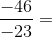Explanation:

The division of two negative numbers yields a positive result.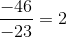### Example Question #101 : Negative Numbers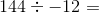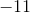Explanation:

In a division problem, if the signs are different (one is negative and one is positive), the answer is negative.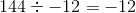### Example Question #101 : Concepts

The temperature has been falling an average of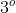per hour.  If the temperature at 1:00 A.M. is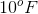, then what will be the weather at 6:00 A.M.?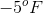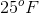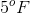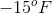Explanation:

If the temperature is droppingforhours (1:00 A.M.-6:00 A.M.), then that would be represented as: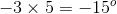If the temperature at 1:00 A.M. is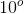then: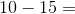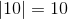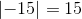Subtract and take the sign of the higher absolute value.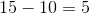The sign of the higher absolute value, which is, is negative.

The temperature isat 6:00 A.M. is the correct answer.

### Example Question #102 : Concepts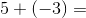Explanation:

The rules for adding integers with different sign is to subtract the absolute value of these numbers, and then take the sign of the higher of the two numbers.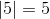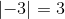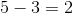Becausehas the larger absolute value and is positive, the correct answer is:.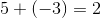### Example Question #103 : Concepts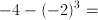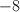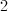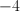Explanation:

The order of operations is PEMDAS, or (1) Parentheses (2) Exponents (3)Multiplication/Division (4) Addition/Subtraction.

First, we need to cube the quantity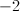.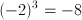Now we are left with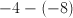.

Subtracting a negative is the same as adding a positive. So we can rewrite this as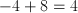### Example Question #1 : Decimals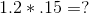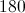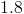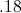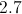Explanation:

When multiplying decimals you ignore the decimals and multiply the two numbers like you would normally.

Then count the total number of decimal places to the right of the decimal of the original numbers.

Move the decimal from the whole number answer you just received to the left the same number of times as the total decimal places to the right of the numbers before you multiplied them.

Therefore the answer is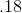### Example Question #1 : Decimals

Divide: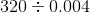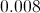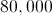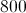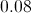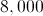Explanation:

Examine the divisor 0.004. To make this a whole number, the decimal point needs to be moved three places right. Do this to both 0.004 and 320, adding an implied decimal point after the latter first: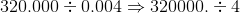Now perform this division: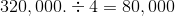### Example Question #2 : Decimals

Evaluate: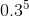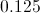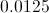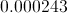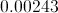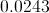Explanation: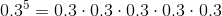First, multiply, ignoring the decimal points: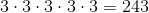Put a decimal point after the number: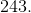Since there were five digits total (one per factor) left of the decimal points, move the decimal point in the product left five places, inserting zeroes as needed: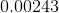### Example Question #2 : Decimals

Express 1.14 as a fraction in lowest terms.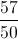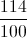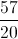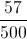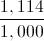Explanation:

The fraction has its last nonzero digit in the hundredths place, so write the number, without the decimal point, over 100. Then reduce.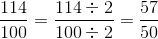### Example Question #1 : Decimals

Express 450,000,000,000 in scientific notation.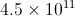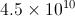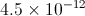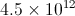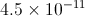Explanation:

To rewrite a very large number in scientific notation:

Write the number with the decimal point after it (it is implied to be at that location).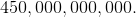Move the decimal point left as many places as needed until it follows the first nonzero digit, which here is the four. Count the number of places it is moved - here it will be eleven places.

The number formed is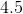, which will be placed in front; 11, the number of places counted, will be the exponent. The number, in scientific notation, will be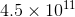.

### All HSPT Math Resources